## Example Questions

← Previous 1

### Example Question #1 : How To Find Circumference

If a circle has an area of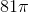, what is the circumference of the circle?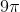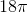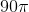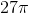Explanation:

The formula for  the area of a circle is πr2. For this particular circle, the area is 81π, so 81π = πr2. Divide both sides by π and we are left with r2=81. Take the square root of both sides to find r=9. The formula for the circumference of the circle is 2πr = 2π(9) = 18π. The correct answer is 18π.

### Example Question #3 : How To Find Circumference

A circle with an area of 13π in2 is centered at point C. What is the circumference of this circle?

√13π

26π

2√13π

13π

√26π

2√13π

Explanation:

The formula for the area of a circle is πr2.

We are given the area, and by substitution we know that 13π πr2.

We divide out the π and are left with 13 = r2.

We take the square root of r to find that r = √13.

We find the circumference of the circle with the formula = 2πr.

We then plug in our values to find = 2√13π.

### Example Question #1 : How To Find Circumference

A 6 by 8 rectangle is inscribed in a circle. What is the circumference of the circle?

12π

10π

25π

8π

6π

10π

Explanation:

First you must draw the diagram. The diagonal of the rectangle is also the diameter of the circle. The diagonal is the hypotenuse of a multiple of 2 of a 3,4,5 triangle, and therefore is 10.
Circumference = π * diameter = 10π.

### Example Question #1 : How To Find Circumference

A gardener wants to build a fence around their garden shown below. How many feet of fencing will they need, if the length of the rectangular side is 12 and the width is 8?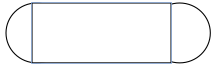8π + 24

4π + 24

96 ft

40 ft.

8π + 24

Explanation:

The shape of the garden consists of a rectangle and two semi-circles. Since they are building a fence we need to find the perimeter. The perimeter of the length of the rectangle is 24. The perimeter or circumference of the circle can be found using the equation C=2π(r), where r= the radius of the circle. Since we have two semi-circles we can find the circumference of one whole circle with a radius of 4, which would be 8π.

### Example Question #1 : How To Find Circumference

The diameter of a circle is defined by the two points (2,5) and (4,6). What is the circumference of this circle?

π√2.5

2.5π

π√5

π√5

Explanation:

We first must calculate the distance between these two points. Recall that the distance formula is:√((x2 - x1)2 + (y2 - y1)2)

For us, it is therefore: √((4 - 2)2 + (6 - 5)2) = √((2)2 + (1)2) = √(4 + 1) = √5

If d = √5, the circumference of our circle is πd, or π√5.

### Example Question #5 : How To Find Circumference

A car tire has a radius of 18 inches. When the tire has made 200 revolutions, how far has the car gone in feet?

3600π

600π

300π

500π

600π

Explanation:

If the radius is 18 inches, the diameter is 3 feet. The circumference of the tire is therefore 3π by C=d(π). After 200 revolutions, the tire and car have gone 3π x 200 = 600π feet.

### Example Question #1 : How To Find Circumference

A circle has the equation below. What is the circumference of the circle?

(x – 2)2 + (y + 3)2 = 9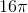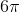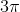Explanation:

The radius is 3. Yielding a circumference of.

### Example Question #2 : How To Find Circumference

What is the circumference of a cirle with a radius of seven?

Leave your answer in terms of.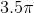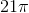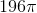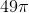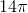Explanation:

Plug the radius into the circumference formula: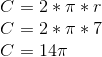### Example Question #3 : How To Find Circumference

A circle has an area of. Using this information find the circumference of the circle.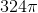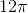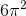Explanation:

To find the circumference of a circle we use the formula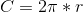.

In order to solve we must use the given area to find the radius. Area of a circle has a formula of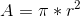.

So we manipulate that formula to solve for the radius.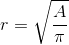.

Then we plug in our given area.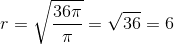.

Now we plug our radius into the circumference equation to get the final answer.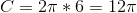.

### Example Question #4 : How To Find Circumference

A circle has an area of. Using this information find the circumference of the circle.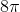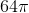Explanation:

To find the circumference of a circle we use the formula.

In order to solve we must use the given area to find the radius. Area of a circle has a formula of.

So we manipulate that formula to solve for the radius..

Then we plug in our given area.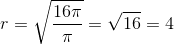.

Now we plug our radius into the circumference equation to get the final answer.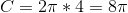.

← Previous 1